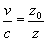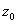Chapter 21

` `

There are two kinds wave within the electromagnetic field, one is the field wave, and the other is the charge density wave.

According to the Gauss Law for both electric field and magnetic field, the electric charge is the source of electric field, and the magnetic charge is the source of the magnetic field.

When an electric current flows through a wire, it creates the electromagnetic field both inside and outside the wire.

Outside the electric wire is a vacuum, both the electric charge density and magnetic density in the vacuum is zero.

When the electric wire has ac current, there exists electromagnetic field wave propagate along the wire.

Inside the electric wire, the electric charge density wave and magnetic charge density charge wave is the source of the electromagnetic field wave.

Outside the wire is a vacuum, which has vacuum impedance. The electromagnetic field wave in the vacuum has light speed.

The electric wire has characteristic electromagnetic impedance. Both the charge density wave and field wave have the same wave velocity. The wave velocity depends on the characteristic impedance of the transmission line. The higher the impedance, the lower the velocity speed. The impedance of the transmission line and velocity of the charge density wave have the reversion relationship:In which ‘v’ is the velocity of charge density sound wave, ‘c’ is the speed of light in vacuum, ‘’ is the impedance of the vacuum, and ‘z’ is the impedance of the transmission line.

The velocity factor is the ratio of the speed of waves in transmission line to the speed of light in the vacuum.

The electromagnetic wave in a transmission line may reflect at the end. If the load is smaller than the line impedance, a reflection will occur with a 180-degree phase shift.

The forwarding wave and reflection of the incoming wave travels back and forth along the wire, which can form a standing wave.

The charge density wave, which involves alternating compression and expansions, similar to a sound wave in the air, is a kind of longitudinal wave.

The charge density wave is a kind of sound wave. The oscillation is in and opposite to the direction of the wave propagation.

The electromagnetic field wave is a kind of transverse wave. The oscillation of its electric field and magnetic field is perpendicular to the direction of propagation of the wave.